Question Pool Tangents

# Tangents - SAMAGRA Question Pool & Answers | Class 10 English Medium

Kerala Syllabus SAMAGRA SCERT SAMAGRA Question Pool for Class 10 English Medium Maths TangentsQn 1.

In the figure PC is the tangent to the circle, If PC = 12 cm , PB = 8 cm and PQ = 2 cm find

a) the length of AP

b) the length of the tangent from Q to C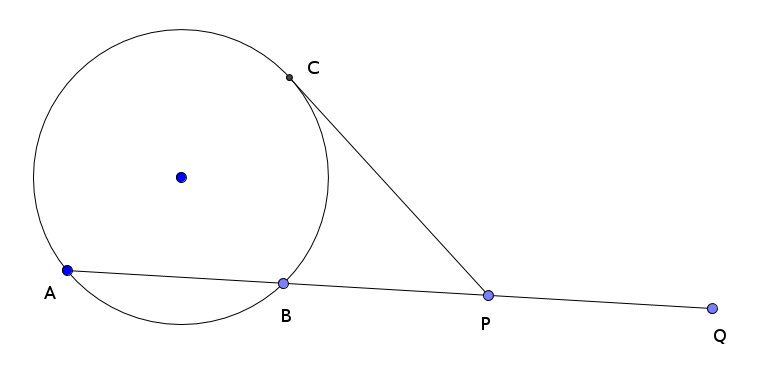Get Free Study Materials + 1 Week Free Trial of BrainsPrep Class 10 English Medium Tuition

Qn 2.

Area of a right triangle is 60 sq. cm. And its inradius is 3cm. Find

a). the perimeter of  the triangle

b). the length of the hypotenuse of the triangle

Get Free Study Materials + 1 Week Free Trial of BrainsPrep Class 10 English Medium Tuition

Qn 3.

In the figure , ABC is a right triangle BP = 3 cm. If the hypotenuse of the triangle is 15 cm. , find

a). the inradius of the circle

b). the perimeter of the triangle

c). the area of the triangle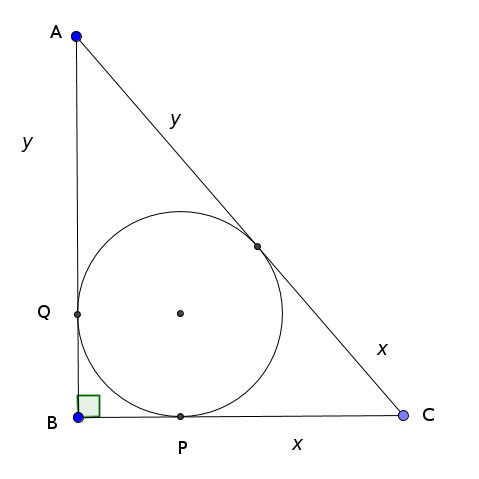r = 3 cm

Get Free Study Materials + 1 Week Free Trial of BrainsPrep Class 10 English Medium Tuition

Qn 4.

Draw a circle of radius 3 cm. Construct an equilateral triangle such that all the sides touching the circle.

Get Free Study Materials + 1 Week Free Trial of BrainsPrep Class 10 English Medium Tuition

Qn 5.

Draw a triangle of sides 8 cm, 7 cm, and 6 cm . Draw its incircle and measure the  inradius.

Get Free Study Materials + 1 Week Free Trial of BrainsPrep Class 10 English Medium Tuition

Qn 6.

The sides of the quadrilateral ABCD touches the circle at P,Q,R and S

a). Find the length of AS

b). Find all the sides of quadrilateral ABCD.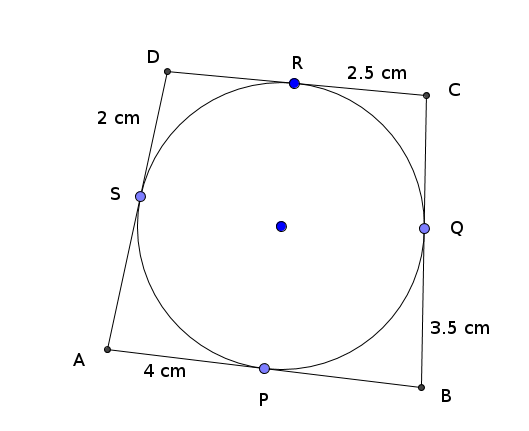AS = AP = 4 cm

For finding AD, AB, BC, CD

Get Free Study Materials + 1 Week Free Trial of BrainsPrep Class 10 English Medium Tuition

Qn 7.

The sides of a triangle are 13 cm, 14 cm and 15 cm.

a). Find the perimeter of the triangle

b) Find Area of the triangle

c) Find Inradius of the triangle

Get Free Study Materials + 1 Week Free Trial of BrainsPrep Class 10 English Medium Tuition

Qn 8.

The perpendicular sides of the right triangle are 9 cm and 12 cm.

a). Find the perimeter of the triangle

b). Find the area of the triangle

c). Find the inradius of the triangle

Get Free Study Materials + 1 Week Free Trial of BrainsPrep Class 10 English Medium Tuition

Qn 9.

In the figure , incircle of  triangle ABC touches the sides of the triangle at P, Q and R. Find the angles of  triangle ABC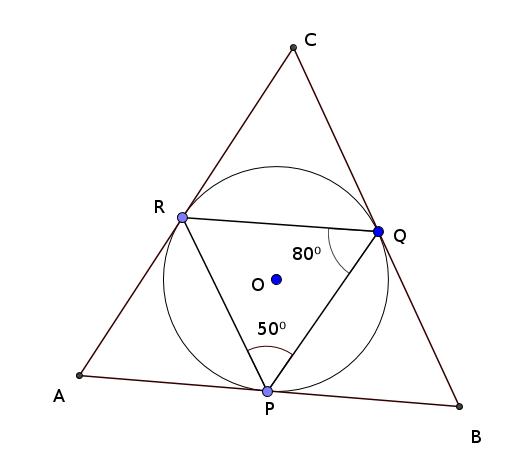∠POR = 2 X 800 = 160

Get Free Study Materials + 1 Week Free Trial of BrainsPrep Class 10 English Medium Tuition

Qn 10.

In the figure O is the centre of the circle and AB is a tangents to the circle. BD = 3.6 cm , CD = 6.4 cm Then

a). find the length of AB

b). find the radius of the circle.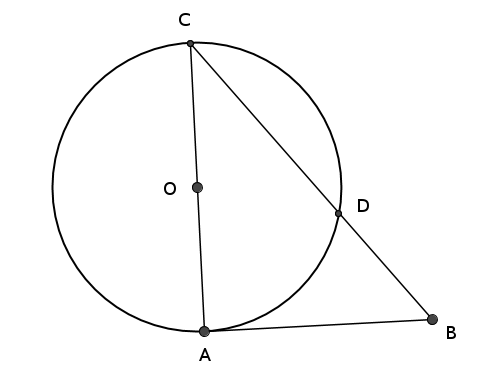BC = 10 cm

Get Free Study Materials + 1 Week Free Trial of BrainsPrep Class 10 English Medium Tuition